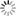# Episode List

OR

### Season 1

18 Oct. 1960Be the first one to add a plot.

25 Oct. 1960
To Coin a CrimeBe the first one to add a plot.

1 Nov. 1960
On ConsignmentIn Geneva, a masked band of thieves steal a valuable coin collection at gunpoint. Robin is put on the case, and finds out among other things , the stolen ducats were fakes.

8 Nov. 1960
Flight to the DesertBe the first one to add a plot.

15 Nov. 1960
The NightmareBe the first one to add a plot.

22 Nov. 1960Be the first one to add a plot.

29 Nov. 1960
The Bard DisappearsBe the first one to add a plot.

6 Dec. 1960
Heart of BuddhaBe the first one to add a plot.

13 Dec. 1960
The PayoffBe the first one to add a plot.

20 Dec. 1960
Zippered NotebookWhen a scientist is knocked unconscious and highly sensitive research papers are stolen in Belgium, Robin must bluff some pointed accusations at all the suspects to force the culprit to action.

26 Dec. 1960
Temporary WindowBe the first one to add a plot.

2 Jan. 1961
The Heartbreak GangBe the first one to add a plot.

9 Jan. 1961
Jungle QuestBe the first one to add a plot.

16 Jan. 1961Be the first one to add a plot.

23 Jan. 1961
Key ManBe the first one to add a plot.

30 Jan. 1961
The TunnelBe the first one to add a plot.

6 Feb. 1961
Spell of DangerBe the first one to add a plot.

13 Feb. 1961
Brink of DisasterBe the first one to add a plot.

20 Feb. 1961
Baubles and BulletsBe the first one to add a plot.

27 Feb. 1961
The Genuine FakeBe the first one to add a plot.

6 Mar. 1961
Policy of MurderBe the first one to add a plot.

13 Mar. 1961Be the first one to add a plot.

20 Mar. 1961
Doll of DeathBe the first one to add a plot.

27 Mar. 1961Be the first one to add a plot.

3 Apr. 1961
Amsterdam LapidaryBe the first one to add a plot.

10 Apr. 1961
Tin CaperIn Hong Kong, Robin must expose a captain who reported a tin shipment lost to collect on the insurance. To gain the crook's confidence he pretend to be a kidnapper and Phyllis his victim.

17 Apr. 1961
The China PassageBe the first one to add a plot.

24 Apr. 1961
The Big BaffleBe the first one to add a plot.

1 May 1961
The Patient DiedBe the first one to add a plot.

8 May 1961
Design for DeathBe the first one to add a plot.

15 May 1961
Wheel of FortuneBe the first one to add a plot.

22 May 1961
A Matter of LifeBe the first one to add a plot.

29 May 1961
JavaBe the first one to add a plot.

5 Jun. 1961
Falling StarBe the first one to add a plot.

12 Jun. 1961
Man on the RunBe the first one to add a plot.

19 Jun. 1961
The Fabulous FlopperBe the first one to add a plot.

26 Jun. 1961
Missing MoneyBe the first one to add a plot.

1 Jul. 1961
La Virgin De Macarena